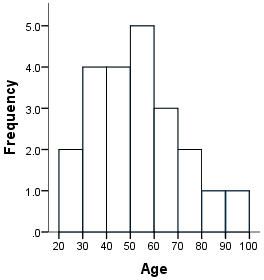Project Management
PMI Sites
Project Management
• Webinars
• Templates
• Community
• Topics
• Knowledge & Tools
• Events
• Other PMI Sites
• PMI Sites

# Histogram

 Contents 1 Applications 2 Procedures 3 Instructions 4 ExamplesA graphical representation of the distribution of numerical data. It is an estimate of the probability distribution of a continuous variable (quantitative variable). Histograms are used to describe the central tendency, dispersion, and shape of a statistical distribution. The histogram does not consider the influence of time on the variation that exists within a distribution.

## Applications

Histograms can be used to show how much variation exists in work, e.g., how much time it takes an organization to complete certain activities. As a picture of the data distribution that includes its spread and shape, a histogram provides clues about the variation that exists in the work performed. Distributions can be skewed in either a positive (tail of the distribution to the right) or negative (tail of the distribution to the left) direction from the center. By examining the spread and shape of a distribution, the extent of variation in a work process can be determined. This can provoke further discussions to identify the cause of variation and the measures needed to either control or reduce it.

A histogram is a useful tool to display the data in order to identify current performance and show how work processes ar improving over time.

## Procedures

Once you have collected a set of data for a repeated activity, complete the following steps.

• 1. Count the number of observations in the data set.
• 2. Determine the data range, subtracting the smallest value from the largest value.
• 3. Decide the number of intervals, displayed as bars, to use.
• 4. Divide the range by the number of intervals. Round the number to a whole number.
• 5. Select the boundaries for each interval so that they are not overlapping.
• 6. Count the number of observations that fall within the boundaries for each interval.
• 7. Draw, scale, and label the horizontal (x-axis) and vertical (y-axis) axis lines of the chart. Label the x-axis for the intervals that cover the data range. Mark the vertical axis from zero until the highest frequency is included.
• 8. Draw vertical bars for each interval. The height of the bars equals the number of observations at that interval. The width of each of the bars should be the same.
• 9. Title the completed chart, describing the nature of the observations being made summarized in the chart and the time frame in which the data was collected.

## Instructions

To construct a histogram, the first step is to "bin" the range of values—that is, divide the entire range of values into a series of intervals—and then count how many values fall into each interval. The bins are usually specified as consecutive, non-overlapping intervals of a variable. The bins (intervals) must be adjacent, and are usually equal size.

If the bins are of equal size, a rectangle is erected over the bin with height proportional to the frequency, the number of cases in each bin. In general, however, bins need not be of equal width; in that case, the erected rectangle has area proportional to the frequency of cases in the bin. The vertical axis is not frequency but density: the number of cases per unit of the variable on the horizontal axis. A histogram may also be normalized displaying relative frequencies. It then shows the proportion of cases that fall into each of several categories, with the sum of the heights equaling 1.

As the adjacent bins leave no gaps, the rectangles of a histogram touch each other to indicate that the original variable is continuous.

## Examples

An example of a histogram, and the raw data it was constructed from, is shown below:Data: 55 42 32 61 54 23 35 71 90 36 42 44 62 55 32 53 51 21 41 63 72 81